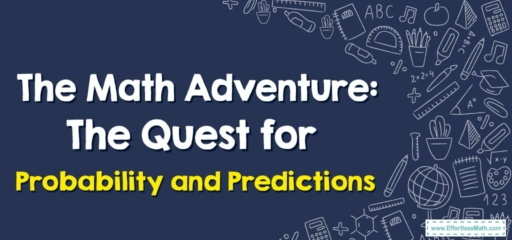# The Math Adventure: The Quest for Probability and Predictions

On today's journey, we're venturing into the magical realm of probability and predictions. We will be wielding our sword of data to slay uncertainties and predict the future. So, pack your gear, and let's embark on this exciting quest!## 1. The Realm of Probability and Predictions

Probability and predictions are magical concepts in mathematics. With the right data, we can predict possible outcomes and determine the likelihood of an event occurring – just like a wizard foreseeing the future!

## 2. The Math Quest: Finding Probabilities and Making Predictions

Our quest involves harnessing the power of collected data to find probabilities and make predictions. But fear not! Here’s a map for our journey:

### The Adventurer’s Map: How to Find Probabilities and Make Predictions Using Collected Data

#### Step 1: Gather Your Data

Our adventure begins with gathering the data – think of it as collecting mystical artifacts for our journey.

#### Step 2: Analyze the Data

Just like deciphering an ancient scroll, we need to understand our data. What does it tell us? What patterns can we observe?

#### Step 3: Calculate Probability

With our data in hand, we can use it to calculate the probability of a particular event. This is done by dividing the number of favorable outcomes by the total number of possible outcomes.

#### Step 4: Make Predictions

Based on our probabilities, we can make predictions about what might happen in the future.

Consider a simple scenario: You’ve collected data about the weather for the past month and noticed that it rained $$7$$ out of $$30$$ days. You want to predict the probability that it will rain tomorrow.

1. Gather Your Data: You’ve recorded weather data for $$30$$ days, noting whether it rained or not each day.
2. Analyze the Data: You observed that it rained $$7$$ days out of the $$30$$.
3. Calculate Probability: The probability of it raining, based on your data, is $$7$$ (rainy days) divided by $$30$$ (total days), which equals approximately $$0.23$$ or $$23$$$$\%$$.
4. Make Predictions: Based on your data and probability calculation, you might predict a $$23$$$$\%$$ chance of rain tomorrow.

And there you have it, brave adventurers! We’ve navigated the mystical realm of probability and predictions. Remember, every data point is a clue to unravel the mysteries of the future. Until our next adventure, stay curious and keep exploring!

### What people say about "The Math Adventure: The Quest for Probability and Predictions - Effortless Math: We Help Students Learn to LOVE Mathematics"?

No one replied yet.

X
30% OFF

Limited time only!

Save Over 30%

SAVE $5 It was$16.99 now it is \$11.99